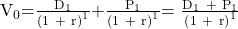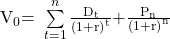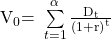Essential Concept 48: Dividend Discount Model (DDM) | IFT World
101 Concepts for the Level I Exam

# Essential Concept 48: Dividend Discount Model (DDM)The value of a stock using DDM for a single-holding period is:The value of a stock using DDM for multiple finite holding periods is:The value of a stock for infinite holding periods is:There are two approaches to forecast dividends.

• One is to assume that they follow a stylized growth pattern (constant growth, two-stages of growth, or three stages of growth).
• The other alternative is to forecast dividends for a finite period, then forecast the remaining dividends based on a pattern or by calculating the terminal stock price.